IMO Shortlist 1993 problem G7

Kvaliteta:
Avg: 0,0
Težina:
Avg: 9,0
Let$A$,$B$,$C$,$D$ be four points in the plane, with$C$ and$D$ on the same side of the line$AB$, such that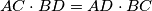$AC \cdot BD = AD \cdot BC$ and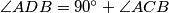$\angle ADB = 90^{\circ}+\angle ACB$. Find the ratio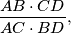and prove that the circumcircles of the triangles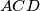$ACD$ and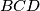$BCD$ are orthogonal. (Intersecting circles are said to be orthogonal if at either common point their tangents are perpendicuar. Thus, proving that the circumcircles of the triangles$ACD$ and$BCD$ are orthogonal is equivalent to proving that the tangents to the circumcircles of the triangles$ACD$ and$BCD$ at the point$C$ are perpendicular.)
Izvor: Međunarodna matematička olimpijada, shortlist 1993